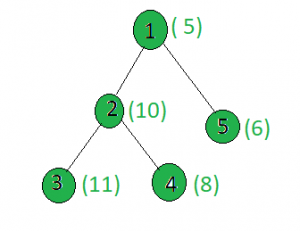# Count the nodes in the given tree whose weight is prime

Given a tree, and the weights of all the nodes, the task is to count the number of nodes whose weight is prime.

Examples:

Input:Output: 2
Only the weights of the nodes 1 and 3 are prime.

## Recommended: Please try your approach on {IDE} first, before moving on to the solution.

Approach: Perform dfs on the tree and for every node, check if it’s weight is prime or not.

Below is the implementation of above approach:

 `// C++ implementation of the approach ` `#include ` `using` `namespace` `std; ` ` `  `int` `ans = 0; ` ` `  `vector<``int``> graph; ` `vector<``int``> weight(100); ` ` `  `// Function that returns true ` `// if n is prime ` `bool` `isprime(``int` `n) ` `{ ` `    ``for` `(``int` `i = 2; i * i <= n; i++) ` `        ``if` `(n % i == 0) ` `            ``return` `false``; ` `    ``return` `true``; ` `} ` ` `  `// Function to perform dfs ` `void` `dfs(``int` `node, ``int` `parent) ` `{ ` `    ``// If weight of node is prime or not ` `    ``if` `(isprime(weight[node])) ` `        ``ans += 1; ` ` `  `    ``for` `(``int` `to : graph[node]) { ` `        ``if` `(to == parent) ` `            ``continue``; ` `        ``dfs(to, node); ` `    ``} ` `} ` ` `  `// Driver code ` `int` `main() ` `{ ` `    ``// Weights of the node ` `    ``weight = 5; ` `    ``weight = 10; ` `    ``weight = 11; ` `    ``weight = 8; ` `    ``weight = 6; ` ` `  `    ``// Edges of the tree ` `    ``graph.push_back(2); ` `    ``graph.push_back(3); ` `    ``graph.push_back(4); ` `    ``graph.push_back(5); ` ` `  `    ``dfs(1, 1); ` ` `  `    ``cout << ans; ` ` `  `    ``return` `0; ` `} `

Output:

```2
```

My Personal Notes arrow_drop_up